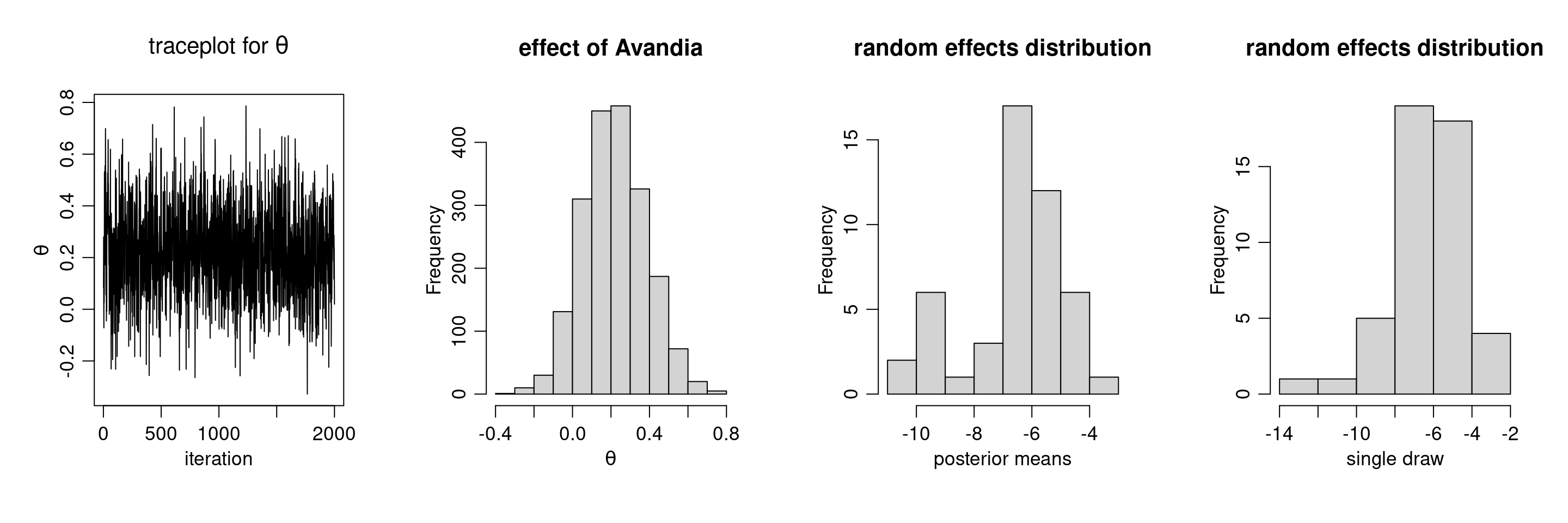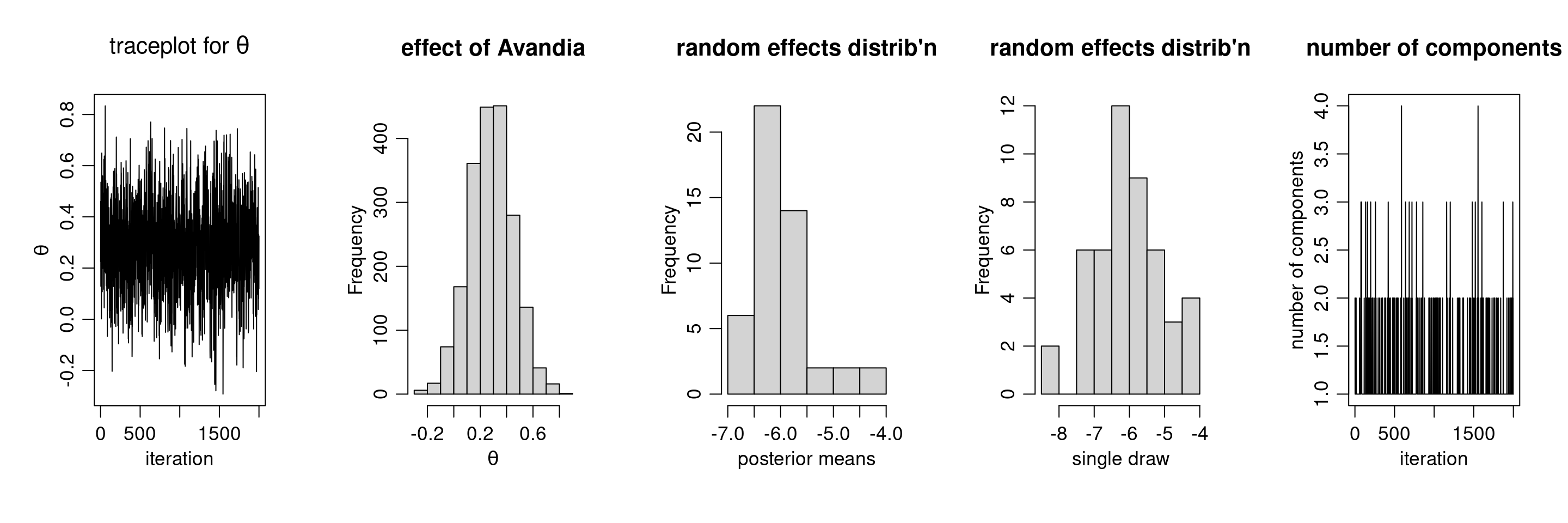# Bayesian nonparametrics in NIMBLE: Nonparametric random effects

NIMBLE provides functionality for fitting models involving Dirichlet process priors using either the Chinese Restaurant Process (CRP) or a truncated stick-breaking (SB) representation of the Dirichlet process prior.

Here we will take a parametric generalized linear mixed model and show how to switch to a nonparametric representation of the random effects that avoids the assumption of normally-distributed random effects.

## Parametric meta analysis of Avandia myocardial infarctions (MIs)

We will illustrate the use of nonparametric mixture models for modeling random effects distributions in the context of a meta-analysis of the side effects of a formerly very popular drug for diabetes called Avandia. The data we analyze played a role in raising serious questions about the safety of this drug. The question is whether Avandia use increases the risk of myocardial infarction (heart attack). There are 48 studies (the 49th study in the data file is different in some ways and excluded here), each with treatment and control arms.

dat <- read.csv('https://r-nimble.org/nimbleExamples/avandia.csv')
head(dat)
##   trial nAvandia avandiaMI nControl controlMI
## 1     1      357         2      176         0
## 2     2      391         2      207         1
## 3     3      774         1      185         1
## 4     4      213         0      109         1
## 5     5      232         1      116         0
## 6     6       43         0       47         1
dat <- dat[-49, ]

### Model formulation

We begin with a standard generalized linear mixed model (GLMM)-based meta analysis. The vectors $$n$$ and $$x$$ contain the total number of patients in the control and the number of patients suffering from myocardial infarctions in the control group of each study, respectively. Similarly, the vectors $$m$$ and $$y$$ contain similar information for patients receiving the drug Avandia. The model takes the form

$x_{i} \mid \theta, \gamma_i \sim \mbox{Bin} \left(n_i, \frac{\exp\left\{ \gamma_i \right\}}{1 + \exp\left\{ \gamma_i \right\}} \right) , \quad\quad y_{i} \mid \theta, \gamma_i \sim \mbox{Bin} \left(m_i, \frac{\exp\left\{ \theta + \gamma_i \right\}}{1 + \exp\left\{ \theta + \gamma_i \right\}} \right)$

where the random effects, $$\gamma_i$$, follow a common normal distribution, $$\gamma_i \sim \mbox{N}(0, \tau^2)$$, and the $$\theta$$ and $$\tau^2$$ are given reasonably non-informative priors. The parameter $$\theta$$ quantifies the difference in risk between the control and treatment arms, while the $$\gamma_i$$ quantify study-specific variation.

This model can be specified in NIMBLE using the following code:

library(nimble, warn.conflicts = FALSE)
## nimble version 1.0.1 is loaded.
##
## Note for advanced users who have written their own MCMC samplers:
##   As of version 0.13.0, NIMBLE's protocol for handling posterior
##   predictive nodes has changed in a way that could affect user-defined
##   samplers in some situations. Please see Section 15.5.1 of the User Manual.
x <- dat$controlMI n <- dat$nControl
y <- dat$avandiaMI m <- dat$nAvandia

nStudies <- nrow(dat)
data <- list(x = x, y = y)
constants = list(n = n, m = m, nStudies = nStudies)

codeParam <- nimbleCode({
for(i in 1:nStudies) {
y[i] ~ dbin(size = m[i], prob = q[i]) # avandia MIs
x[i] ~ dbin(size = n[i], prob = p[i]) # control MIs
q[i] <- expit(theta + gamma[i])       # Avandia log-odds
p[i] <- expit(gamma[i])               # control log-odds
gamma[i] ~ dnorm(mu, var = tau2)      # study effects
}
theta ~ dflat()        # effect of Avandia
# random effects hyperparameters
mu ~ dnorm(0, 10)
tau2 ~ dinvgamma(2, 1)
})

### Running the MCMC

Let’s run a basic MCMC.

set.seed(9)
inits = list(theta = 0, mu = 0, tau2 = 1, gamma = rnorm(nStudies))

samples <- nimbleMCMC(code = codeParam, data = data, inits = inits,
constants = constants, monitors = c("mu", "tau2", "theta", "gamma"),
thin = 10, niter = 22000, nburnin = 2000, nchains = 1,
setSeed = TRUE)
## Defining model
## Building model
## Setting data and initial values
## Running calculate on model
##   [Note] Any error reports that follow may simply reflect missing values in model variables.
## Checking model sizes and dimensions
## Checking model calculations
## Compiling
##   [Note] This may take a minute.
##   [Note] Use 'showCompilerOutput = TRUE' to see C++ compilation details.
## running chain 1...
## |-------------|-------------|-------------|-------------|
## |-------------------------------------------------------|
par(mfrow = c(1, 4), cex = 1.1, mgp = c(1.8,.7,0))
ts.plot(samples[ , 'theta'], xlab = 'iteration', ylab = expression(theta),
main = expression(paste('traceplot for ', theta)))
hist(samples[ , 'theta'], xlab = expression(theta), main = 'effect of Avandia')

gammaCols <- grep('gamma', colnames(samples))
gammaMn <- colMeans(samples[ , gammaCols])
hist(gammaMn, xlab = 'posterior means', main = 'random effects distribution')
hist(samples[1000, gammaCols], xlab = 'single draw',
main = 'random effects distribution')The results suggests there is an overall difference in risk between the control and treatment arms. But what about the normality assumption? Are our conclusions robust to that assumption? Perhaps the random effects distribution are skewed. (And recall that the estimates above of the random effects are generated under the normality assumption, which pushes the estimated effects to look more normal…)

## DP-based random effects modeling for meta analysis

### Model formulation

Now, we use a nonparametric distribution for the $$\gamma_i$$s. More specifically, we assume that each $$\gamma_i$$ is generated from a location-scale mixture of normal distributions:

$\gamma_i \mid \mu_i, \tau_i^2 \sim \mbox{N}(\mu_i, \tau_i^2), \quad\quad (\mu_i, \tau_i^2) \mid G \sim G, \quad\quad G \sim \mbox{DP}(\alpha, H),$

where $$H$$ is a normal-inverse-gamma distribution.

This specification induces clustering among the random effects. As in the case of density estimation problems, the DP prior allows the data to determine the number of components, from as few as one component (i.e., simplifying to the parametric model), to as many as $$n$$ components, i.e., one component for each observation. This allows the distribution of the random effects to be multimodal if the data supports such behavior, greatly increasing its flexibility. This model can be specified in NIMBLE using the following code:

codeBNP <- nimbleCode({
for(i in 1:nStudies) {
y[i] ~ dbin(size = m[i], prob = q[i])   # avandia MIs
x[i] ~ dbin(size = n[i], prob = p[i])   # control MIs
q[i] <- expit(theta + gamma[i])         # Avandia log-odds
p[i] <- expit(gamma[i])                 # control log-odds
gamma[i] ~ dnorm(mu[i], var = tau2[i])  # random effects from mixture dist.
mu[i] <- muTilde[xi[i]]                 # mean for random effect from cluster xi[i]
tau2[i] <- tau2Tilde[xi[i]]             # var for random effect from cluster xi[i]
}
# mixture component parameters drawn from base measures
for(i in 1:nStudies) {
muTilde[i] ~ dnorm(mu0, var = var0)
tau2Tilde[i] ~ dinvgamma(a0, b0)
}
# CRP for clustering studies to mixture components
xi[1:nStudies] ~ dCRP(alpha, size = nStudies)
# hyperparameters
alpha ~ dgamma(1, 1)
mu0 ~ dnorm(0, 10)
var0 ~ dinvgamma(2, 1)
a0 ~ dinvgamma(2, 1)
b0 ~ dinvgamma(2, 1)
theta ~ dflat()          # effect of Avandia
})

### Running the MCMC

The following code compiles the model and runs a collapsed Gibbs sampler for the model

inits <- list(gamma = rnorm(nStudies), xi = sample(1:2, nStudies, replace = TRUE),
alpha = 1, mu0 = 0, var0 = 1, a0 = 1, b0 = 1, theta = 0,
muTilde = rnorm(nStudies), tau2Tilde = rep(1, nStudies))

samplesBNP <- nimbleMCMC(code = codeBNP, data = data, inits = inits,
constants = constants,
monitors = c("theta", "gamma", "alpha", "xi", "mu0", "var0", "a0", "b0"),
thin = 10, niter = 22000, nburnin = 2000, nchains = 1, setSeed = TRUE)
## Defining model
## Building model
## Setting data and initial values
## Running calculate on model
##   [Note] Any error reports that follow may simply reflect missing values in model variables.
## Checking model sizes and dimensions
## Checking model calculations
## Compiling
##   [Note] This may take a minute.
##   [Note] Use 'showCompilerOutput = TRUE' to see C++ compilation details.
## running chain 1...
## |-------------|-------------|-------------|-------------|
## |-------------------------------------------------------|
gammaCols <- grep('gamma', colnames(samplesBNP))
gammaMn <- colMeans(samplesBNP[ , gammaCols])
xiCols <- grep('xi', colnames(samplesBNP))

par(mfrow = c(1,5), cex = 1.1, mgp = c(1.8,.7,0))
ts.plot(samplesBNP[ , 'theta'], xlab = 'iteration', ylab = expression(theta),
main = expression(paste('traceplot for ', theta)))
hist(samplesBNP[ , 'theta'], xlab = expression(theta), main = 'effect of Avandia')
hist(gammaMn, xlab = 'posterior means',
main = "random effects distrib'n")
hist(samplesBNP[1000, gammaCols], xlab = 'single draw',
main = "random effects distrib'n")

# How many mixture components are inferred?
xiRes <- samplesBNP[ , xiCols]
nGrps <- apply(xiRes, 1, function(x) length(unique(x)))
ts.plot(nGrps, xlab = 'iteration', ylab = 'number of components',
main = 'number of components')The primary inference seems robust to the original parametric assumption. This is probably driven by the fact that there is not much evidence of lack of normality in the random effects distribution (as evidenced by the fact that the posterior distribution of the number of mixture components places a large amount of probability on exactly one component).